[Next]: Homoclinic bifurcations
 [Up]: Project descriptions
 [Previous]: Dynamics of semiconductor lasers
[Contents]   [Index]

Subsections

## Multiscale systems

Collaborator: K.R. Schneider , E.V. Shchetinina

#### Project 1: Exchange of stabilities in multiscale systems.

Cooperation with: V.F. Butuzov, N.N. Nefedov (Moscow State University, Russia)

Supported by: DFG: Cooperation Project Singulär gestörte Systeme und Stabilitätswechsel'' (Singularly perturbed systems and exchange of stability) of German and Russian scientists in the framework of the Memorandum of Understanding between DFG and RFFI

Description:

The problem of delayed exchange of stabilities for a scalar non-autonomous ordinary differential equation has been treated some time ago (). Very recently, the same problem for the scalar singularly perturbed parabolic differential equation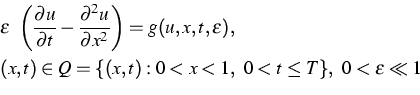(1)

has been solved successfully by means of the method of asymptotic lower and upper solutions . This result provides a first proof for the existence of canard solutions for partial differential equations.

Under the assumption that the degenerate equation

g(u,x,t,0) =0

has exactly two roots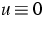and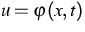intersecting in some smooth curve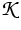with the representation t=tc (x),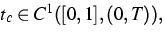and that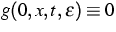for all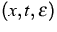under consideration, we derive conditions on g and on u0 such that the initial-boundary value problem to (1)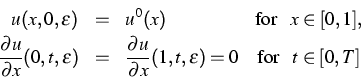has for sufficiently small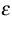a unique solution satisfying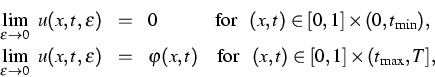that is,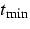and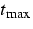are lower and upper bounds for the delay of exchange of stabilities.

The results of the research cooperation between WIAS and the Department of Mathematics of the Faculty of Physics of the Moscow State University in the field of exchange of stabilities in multiscale systems over the last five years have been represented as a report  which will appear as a research monography in Russia and in the USA.

References:

1. V.N. BUTUZOV, N.N. NEFEDOV, K.R. SCHNEIDER, Singularly perturbed problems in case of exchange of stabilities, WIAS Report no. 21 , 2002.
2. N.N. NEFEDOV, K.R. SCHNEIDER, Delayed exchange of stabilities in a class of singularly perturbed parabolic problems, WIAS Preprint no. 778 , 2002.
3.,Delayed exchange of stabilities in singularly perturbed systems, WIAS Preprint no. 270 , 1997, Z. Angew. Math. Mech., 78 (1998), Suppl. 1, S199-S202.

#### Project 2: Delayed loss of stability in non-autonomous delay-differential equations

Cooperation with: B. Lani-Wayda (Justus-Liebig-Universität Giessen)

Description: Dynamical systems as mathematical models of real-life processes depend on several parameters which are assumed to be fixed within some time period. The influence of parameters on the behavior of a dynamical system is studied within the framework of bifurcation theory. This project aims to study the influence of some relevant system parameter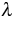changing slowly in time (for example, because of an aging process). For ordinary differential equations it is well known that a slowly changing parameter can lead to a special phenomenon known as delayed loss of stability . Such phenomenon manifests in a jumping behavior of some state variables which can imply dramatic consequences (e.g., thermal explosion). The main goal of this project is to describe a similar effect for nonlinear differential-delay equations of the type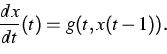(2)

For this purpose, we study the linear inhomogeneous equation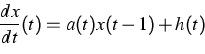(3)

assuming that the function a takes values in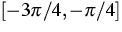and changes slowly. It is well known that for constant a and h = 0, the zero solution of the linear equation (3) is stable for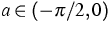,and unstable for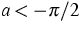. Contrary to the ODE case, the exponential rate of growth or decay is not directly given by a, but has to be estimated. We provide such estimates and derive a variation-of-constants formula for the case of nonconstant a and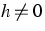. This formula will be used to express solutions of (2) on successive time intervals Ii by solutions of the equation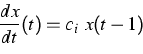with constants ci which are values of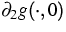on Ii. We establish estimates that express the phenomenon of delayed loss of stability for differential-delay equations of type (2). As an example we treat the equation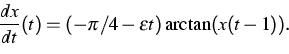Here, we study the initial value problem with the initial segment identically 1, and estimate the time until the solution is close enough to zero by a method that is not based on linearization. Figure 1 demonstrates this phenomenon numerically.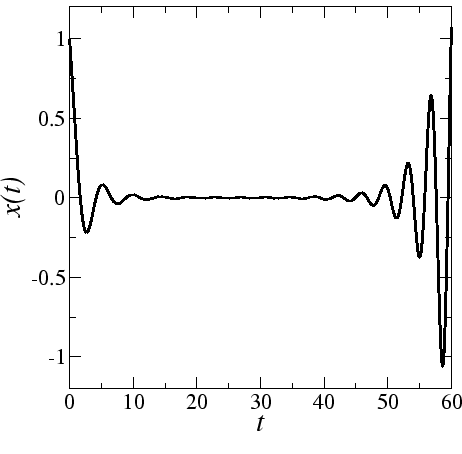Complementary to the results on delayed loss of stability, which express similar behavior of delay equations and ordinary differential equations (ODEs), we exhibit a substantial difference between both types of equations. Namely, the additive term h(t) in the equation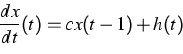inevitably has an influence on the development of all components'' of solutions (in terms of expansion into eigenfunctions of the homogeneous equation). Of course, for a linear constant coefficient system of ODEs, the perturbation h can be chosen such that it influences only specific components.

References:

1. B. LANI-WAYDA, K.R. SCHNEIDER, Delayed loss of stability and excitation of oscillations in nonautonomous differential equations with retarded argument, WIAS Preprint no. 744 , 2002.
2. N.N. NEFEDOV, K.R. SCHNEIDER, Delayed exchange of stabilities in singularly perturbed systems, WIAS Preprint no. 270 , 1997, Z. Angew. Math. Mech., 78 (1998), Suppl. 1, S199-S202.

#### Project 3: Integral manifolds of canard type for non-hyperbolic slow-fast systems

Cooperation with: V.A. Sobolev, E.A. Shchepakina (Samara State University, Russia)

Description: Slow-fast systems have been considered which can be transformed into the form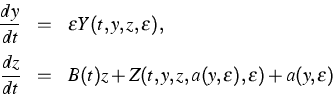(4)

with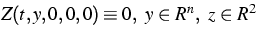,is a small positive parameter, a is a 2-dimensional vector function, and B(t) is the matrix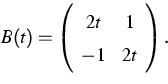Note that the eigenvalues of the matrix B(t) are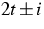, that is, B(0) has purely imaginary eigenvalues, and therefore (4) is a non-hyperbolic slow-fast system.

The aim of this project is to establish an integral manifold of the form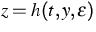to reduce the order of system (4) under non-hyperbolicity conditions. In the hyperbolic case the existence of such integral manifolds has been known for a long time (see, e.g., ).

As a result we derived conditions on Y and Z guaranteeing the existence of a function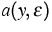such that (4) has an integral manifold of the form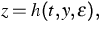where h is uniformly bounded, ||h|| and ||a|| tend to zero as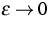.Moreover, we derived an algorithm to determine the coefficients in the asymptotic representation of the functionsand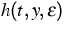as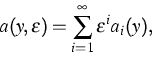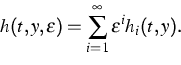The established manifoldis of canard type, that is, it is attracting for t<0 and repelling for t>0.

References:

1. K.R. SCHNEIDER, E.V. SHCHETININA, One-parametric families of canard cycles: Two explicitly solvable examples, to appear in: Z. Angew. Math. Mech.
2. E.V. SHCHETININA, Existence and asymptotic expansion of invariant manifolds for non-hyperbolic slow-fast systems, WIAS Preprint, in preparation.
3. V.V. STRYGIN, V.A. SOBOLEV, Separation of Motions by the Integral Manifold Method (in Russian), Nauka, Moscow, 1988.

#### Project 4: Combustion wave of canard type

Cooperation with: V.A. Sobolev, E.A. Shchepakina (Samara State University, Russia)

Description:

We consider the problem of thermal explosion in case of an autocatalytic combustion reaction. The goal of the project is to establish the existence of traveling wave solutions of the system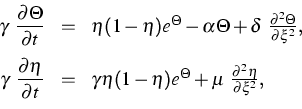(5)

where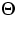is the temperature,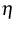the depth of conversion of the gas mixture,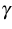is a small parameter (case of highly exothermic reaction). The existence of a traveling wave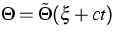,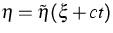to (5) is equivalent to the existence of a heteroclinic orbit of the system (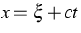)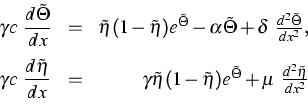(6)

connecting two equilibria of the reaction system.
Basing on the fact that the reaction system has canard solutions separating the slow combustion regime from the explosive one , we prove by applying the geometric theory of singularly perturbed differential equations the existence of a new type of traveling wave solutions, the so-called canard traveling waves.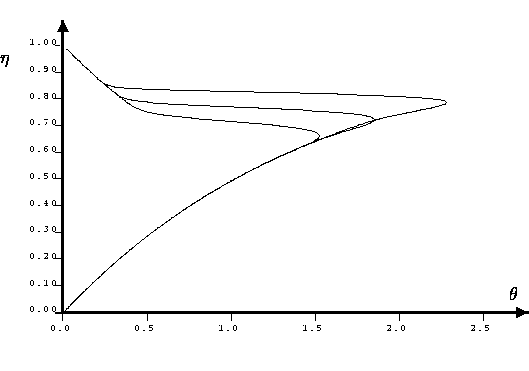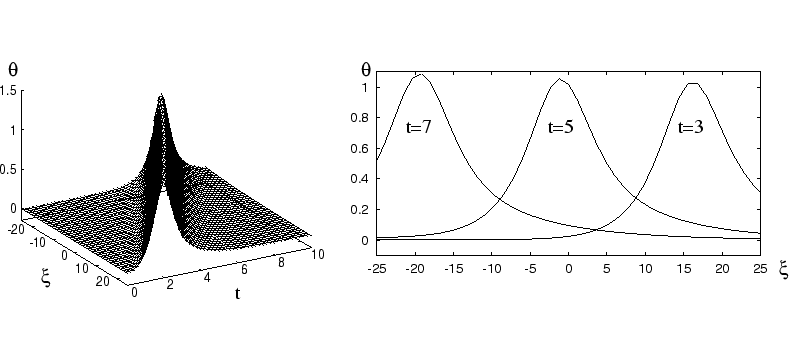References:

1. K.R. SCHNEIDER, V.A. SOBOLEV, Existence and approximation of slow integral manifolds in some degenerate cases, WIAS Preprint no. 782 , 2002.
2. V.A. SOBOLEV, E.A. SHCHEPAKINA, Duck trajectories in a problem of combustion theory, Differential Equations, 32 (1996), pp. 1177-1186.
3. K.R. SCHNEIDER, E.A. SHCHEPAKINA, V.A. SOBOLEV, A new type of traveling wave solutions, WIAS Preprint no. 694 , 2001, to appear in: Math. Methods Appl. Sci.

 [Next]: Homoclinic bifurcations
 [Up]: Project descriptions
 [Previous]: Dynamics of semiconductor lasers
[Contents]   [Index]

LaTeX typesetting by I. Bremer
5/16/2003